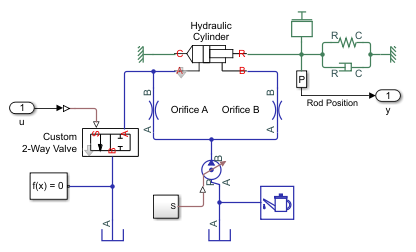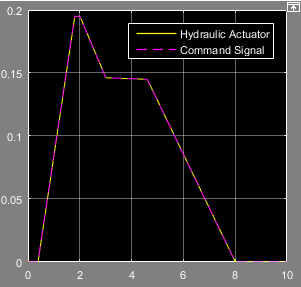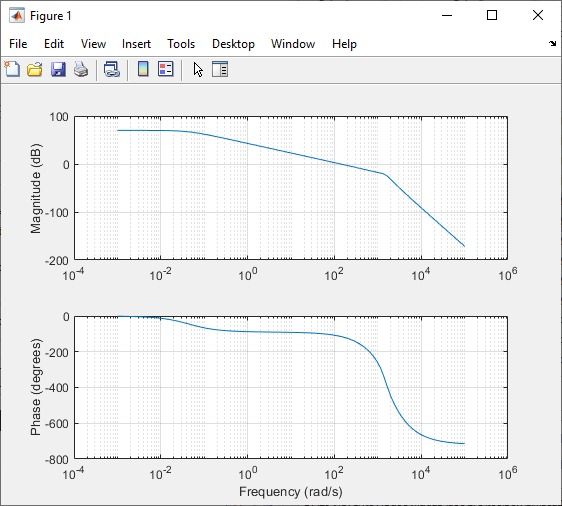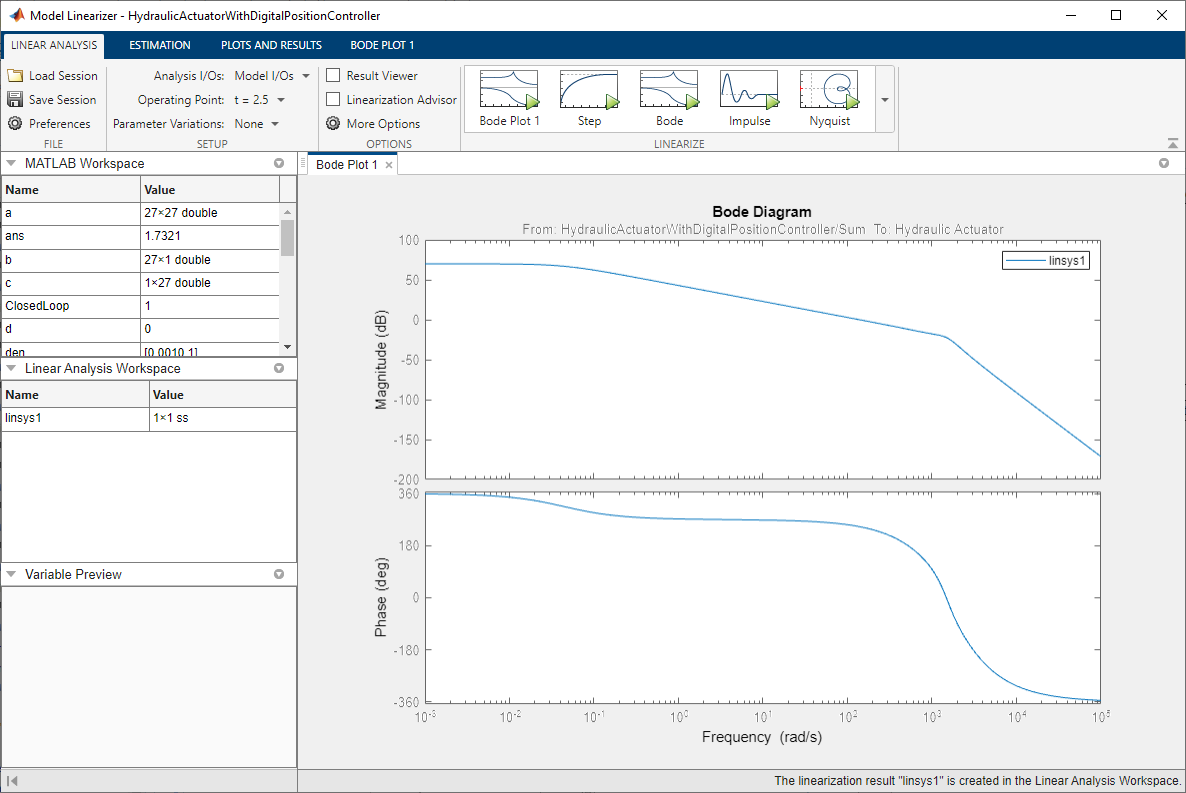## Linearize a Plant Model for Use in Feedback Control Design

This example shows how you can linearize a hydraulic plant model to support control system stability analysis and design.

Depending on the software you have available, use the appropriate sections of this example to explore various linearization and analysis techniques.

### Explore the Model

To open the Hydraulic Actuator with Digital Position Controller example model, type `ssc_hydraulic_actuator_digital_control` in the MATLAB® Command Window.The model represents a two-way valve acting in a closed-loop circuit together with a double-acting cylinder. Double-click the Hydraulic Actuator subsystem to see the model configuration.The controller is represented as a continuous-time transfer function plus a transport delay that allows for computational time and a zero-order hold when implemented in discrete time. The Linearization I/O points subsystem lets you easily break and restore the feedback control loop by setting the base workspace variable ClosedLoop to 0 or 1, respectively.You can quickly generate and view the small-signal frequency response by clicking the `Linearize` hyperlink in model annotation. To view the MATLAB script that generates the frequency response, click the next hyperlink in that annotation, `see code`. This documentation provides background information and alternative ways of linearization based on the software you have.

In general, to obtain a nontrivial linearized input-output model and generate a frequency response, you must specify model-level inputs and outputs. The Hydraulic Actuator with Digital Position Controller model meets this requirement in two ways, depending on how you linearize:

• Simulink® requires top- or model-level input and output ports for linearization with `linmod`. The model has such ports, marked `In1` and `Out1`.

• Simulink Control Design™ software requires that you specify input and output signal lines with linearization points. The specified lines must be Simulink signal lines, not Simscape™ physical connection lines. The model has such linearization points specified. For more information on using Simulink Control Design software for trimming and linearization, see documentation for that product.

Open the Load Position scope and simulate the model in a normal closed-loop controller configuration.You can see that the model has a quasi-linear steady-state response between 2 and 3 seconds, when the two-way valve is open. Therefore, the state at 2.5 seconds is an operating point suitable for linearization.

### Trim Using the Controller and Linearize with Simulink`linmod` Function

1. Set the controller parameters.

To specify sample time for controller discrete-time implementation, type the following in the MATLAB Command Window:

`ts = 0.001;`

To specify continuous-time controller numerator and denominator, type:

```num = -0.5; den = [1e-3 1];```
2. Find an operating point by running closed-loop and selecting the state at 2.5 seconds when the custom two-way valve is open.

To close the feedback loop, type:

`assignin('base','ClosedLoop',1);`

To simulate the model and save the operating point information in the form of a state vector X and input vector U, type:

```[t,x,y] = sim('ssc_hydraulic_actuator_digital_control'); idx = find(t>2.5,1); X = x(idx,:); U = y(idx);```
3. Linearize the model using the Simulink `linmod` function.

To break the feedback loop, type:

`assignin('base','ClosedLoop',0);`

To linearize the model, type:

`[a,b,c,d] = linmod('ssc_hydraulic_actuator_digital_control',X,U);`

Close the feedback loop by typing:

`assignin('base','ClosedLoop',1);`
4. To generate a Bode plot with negative feedback convention, type the following in the MATLAB Command Window:

```c = -c; d = -d; npts = 100; w = logspace(-3,5,npts); G = zeros(1,npts); for i = 1:npts G(i) = c*(1i*w(i)*eye(size(a))-a)^-1*b +d; end subplot(211), semilogx(w,20*log10(abs(G))) grid ylabel('Magnitude (dB)') subplot(212), semilogx(w,180/pi*unwrap(angle(G))) ylabel('Phase (degrees)') xlabel('Frequency (rad/s)') grid ```### Linearize with Simulink Control Design Software

Note

To work through this section, you must have a Simulink Control Design license.

Simulink Control Design software has tools that help you find operating points and returns a state-space model object that defines state names. This is the recommended way to linearize Simscape models.

1. In the Simulink Toolstrip of the Hydraulic Actuator with Digital Position Controller model window, on the Apps tab, under Control Systems, click Model Linearizer.

2. In the Model Linearizer window, on the Linear Analysis tab, in the Operating Point drop-down list, select `Linearize At`. Enter simulation snapshot time of `2.5` seconds and click .

3. Click the Bode plot button.